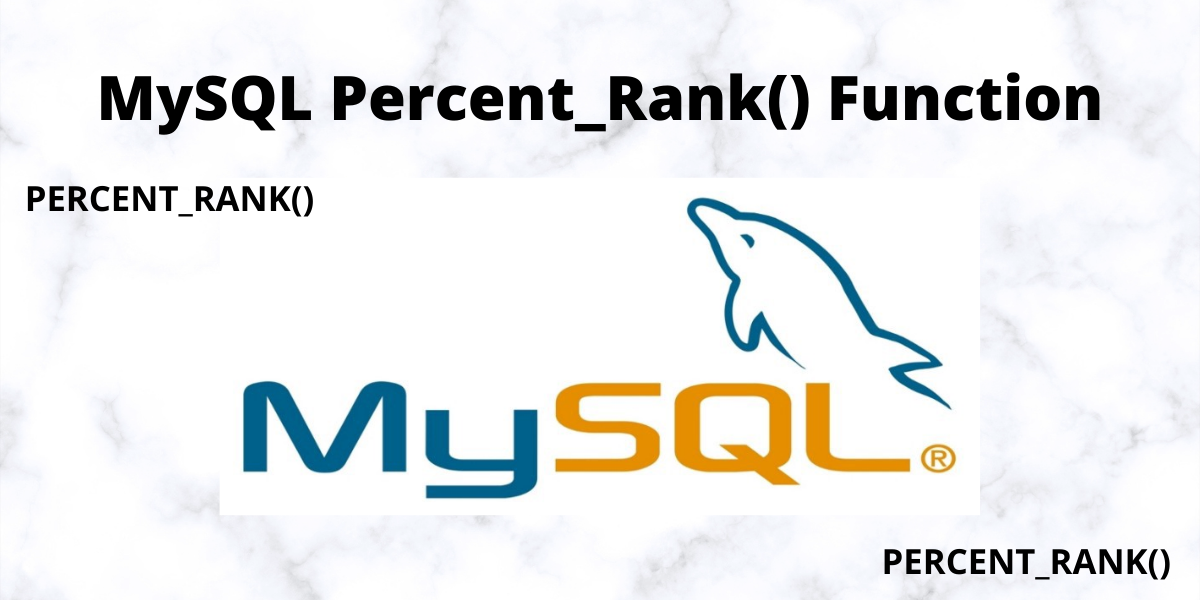# MySQL Percent_Rank() FunctionIn this tutorial, we will study Percent_Rank() function in MySQL. This function takes a column name as the argument and returns the number of rows with greater values than the sample value. Without further ado, let’s get started!

## Introduction to MySQL Percent_Rank()

The Percent_Rank() function is one of many useful functions that are available in MySQL. This function works by taking a single column as an input, and returning the number of rows in that column that contains values that are greater than the value in the current row. In other words, if you have a column with values 1, 2, 3, and 4, and your current row has a value of 3, the Percent_Rank() function would return 0.75, or 3/4.

This function is useful for a 2 main reasons.

• First, it can be used to quickly identify the most popular values in a dataset. If you have hundreds, or even thousands of rows, using Percent_Rank() can help you find the most common values without having to go through every single row manually.
• Second, Percent_Rank() can be used as part of more advanced statistical analyses. For example, if you wanted to determine the correlation between two different columns in a dataset, you could use Percent_Rank() and other functions to calculate the Pearson coefficient of correlation.

In MySQL, the Percent_Rank() function is a window function that is used to calculate the percentile rank of a row within a result set.

The syntax for the following function is-

```.wp-block-code {
border: 0;
}

.wp-block-code > span {
display: block;
overflow: auto;
}

.shcb-language {
border: 0;
clip: rect(1px, 1px, 1px, 1px);
-webkit-clip-path: inset(50%);
clip-path: inset(50%);
height: 1px;
margin: -1px;
overflow: hidden;
position: absolute;
width: 1px;
word-wrap: normal;
word-break: normal;
}

.hljs {
box-sizing: border-box;
}

.hljs.shcb-code-table {
display: table;
width: 100%;
}

.hljs.shcb-code-table > .shcb-loc {
color: inherit;
display: table-row;
width: 100%;
}

.hljs.shcb-code-table .shcb-loc > span {
display: table-cell;
}

.wp-block-code code.hljs:not(.shcb-wrap-lines) {
white-space: pre;
}

.wp-block-code code.hljs.shcb-wrap-lines {
white-space: pre-wrap;
}

.hljs.shcb-line-numbers {
border-spacing: 0;
counter-reset: line;
}

.hljs.shcb-line-numbers > .shcb-loc {
counter-increment: line;
}

.hljs.shcb-line-numbers .shcb-loc > span {
}

.hljs.shcb-line-numbers .shcb-loc::before {
border-right: 1px solid #ddd;
content: counter(line);
display: table-cell;
text-align: right;
-webkit-user-select: none;
-moz-user-select: none;
-ms-user-select: none;
user-select: none;
white-space: nowrap;
width: 1%;
}```PERCENT_RANK()
OVER (
PARTITION BY expr,...
ORDER BY expr [ASC|DESC],...
)```Code language: SQL (Structured Query Language) (sql)```

The result returned by the Percent_Rank() Function ranges from 0 to 1. The formula to calculate the Percent_Rank() function is as follows-

``(rank - 1) / (total_rows - 1)``

The Percent_Rank() function will always return 0 for the first row in the result set.

## Example

Here to illustrate the example of Percent_Rank() function we will use the classicmodels database. A new table named details will be created based on the prior tables named orders, orderDetails, and products. The code for the following is-

``````Create table details
Select
productLine, YEAR(orderDate) OrderYear,
quantityOrdered * priceEach Value
From
orderDetails
Inner Join
orders Using (orderNumber)
Inner Join
products Using (productCode)
Group By
productLine,
YEAR(orderDate);```Code language: SQL (Structured Query Language) (sql)```
``Select * From details;`Code language: SQL (Structured Query Language) (sql)`

Output-

## Query over result set using MySQL Percent_Rank() function

Now we will write a code that will find the percentile rank of every product.

``````With b As (
Select productLine,
Sum(Value) Value
From
details
Group By productLine
)
Select productLine, Value,
Round(
Percent_Rank() OVER (
ORDER BY Value) ,2) percentile_rank
From b;```Code language: SQL (Structured Query Language) (sql)```

Output-

Here we can see that we have calculated the percentile_rank of every product Line.

## MySQL Percent_Rank() with Partition

Now we will write a query that will return the percentile rank of each product line arranged in order with respect to the values.

``````Select
productLine,
orderYear,
Value,
ROUND(
PERCENT_RANK()
OVER (
PARTITION BY orderYear
ORDER By Value),2) percentile_rank
From
details;```Code language: SQL (Structured Query Language) (sql)```

Output-